安森美获选5项负盛名的Elektra奖For too long, we have defined things by their extremes: minimum or maximum, price ranges, or ranges of distances. Often, we tend to ignore the nominal. Instead of always defining things by their extremes, sometimes it makes sense to go by their nominal values. In the WebDesigner+ Power Supply™ tool, we offer the ability to design a power supply considering the nominal voltage operation.Figure 1: "Sometimes it is better to be normal". Caricature drawn by Surinder P. Singh and graphics artwork by Kush Kaur.

A power supply converts voltage from one value to another. The input voltage is typically a range, often centered around the nominal voltage. However, some applications have an asymmetric range around the nominal value. For example, suppose the source voltage has a nominal value of 12 volts. In that case, the power supply designer may design the power supply to work for a range of input voltage of (say) 10 to 14 volts based on the system and application considerations.

Consider the battery-connected automotive power supply design: the car battery has a nominal voltage of 12 volts. Battery voltage during cold crank may drop to 3 or 6 volts. During load dump, the battery voltage may go as high as 28 or 36 volts. These severe extremes are not symmetrical around the nominal voltage. Designers of automotive power systems know that that the extremes are only transient. While a power supply design needs to withstand and function at the extremes, it requires optimization for the nominal voltage. In these scenarios, the WebDesigner+ Power Supply tool can help you select and design for the nominal voltage.

WebDesigner+ Power Supply with Nominal Input Voltage

Let me demonstrate this power supply design in the WebDesigner+ Power Supply tool. Start at the INPUTS tab and enter the input voltage minimum and maximum: set Vin min to 6 volts and Vin max to 36 volts. Click on the accordion labeled “Optional parameters” to expand it, and enter the nominal voltage of 12 volts, as shown in figure 2. You can see that the input voltage range is not symmetrical around the nominal voltage: the lower limit of input is 6 volts below the nominal (12 – 6 = 6 volts), and the higher limit is 24 volts above the nominal (36 – 12 = 24 volts).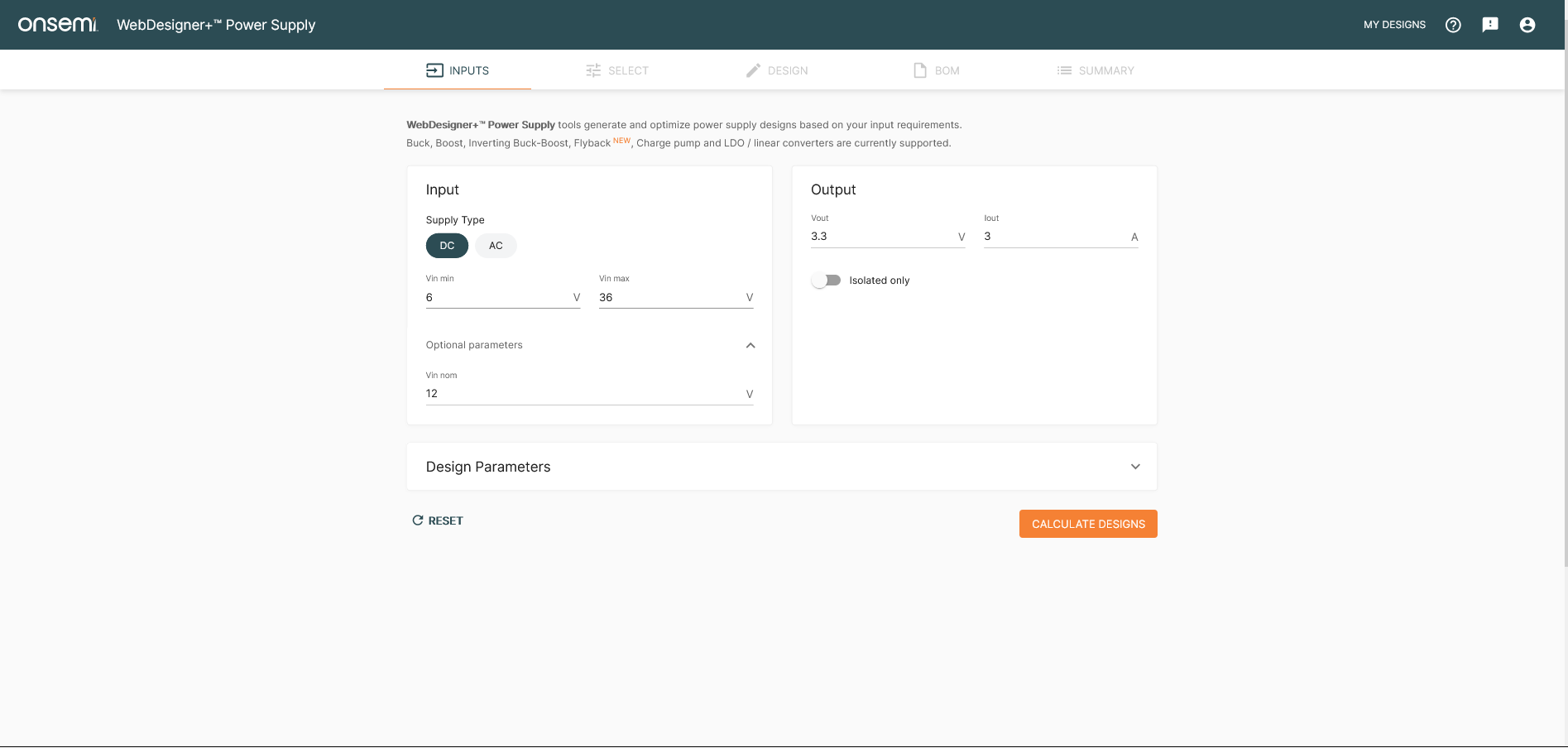Figure 2: INPUT screen of the WebDesigner+ Power Supply tool. The nominal input voltage is entered in the optional parameters area.

Set the output voltage to 3.3 volts at an output current of 3 amperes. Click on the button to “CALCULATE DESIGNS” to go to the SELECT screen, where various power supply solutions are listed, as shown in figure 3. This screen illustrates critical aspects of the design — calculated efficiency and IC junction temperature along with estimated BOM cost, BOM count, and footprint. A note at the bottom of the table, “*Operating values are calculated for nominal input voltage (12 V),” informs the user that all operating values are at the nominal value, not the worst case of the input voltage range.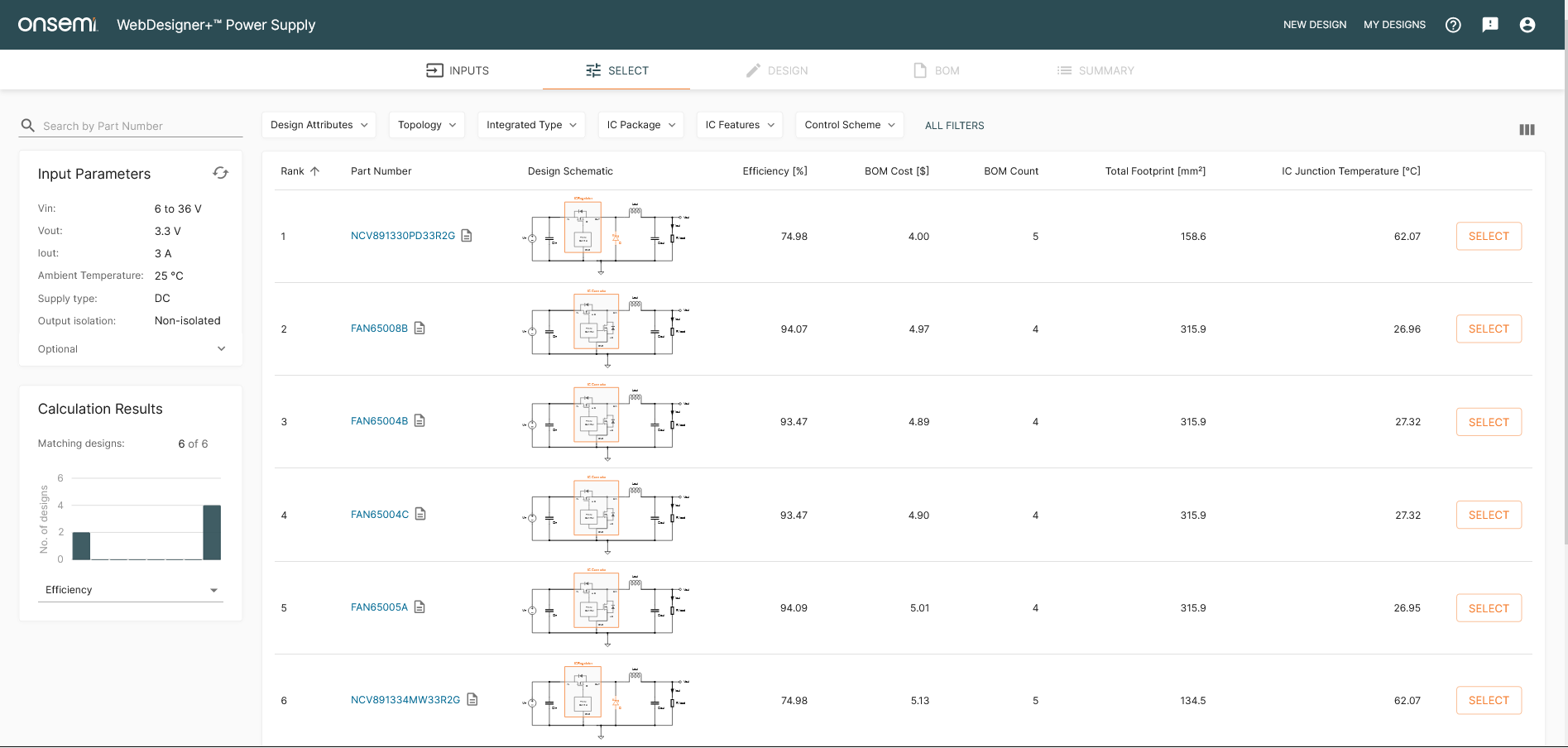Figure 3: SELECT screen of the WebDesigner+ Power Supply tool. All designs which will work for the given input-output conditions are shown. Solutions performance is shown and ranked for the nominal input voltage.

While the listed designs take the minimum and the maximum into account, the design results, selection, and ranking of the products are based on the nominal voltage results. Let’s drill down a little bit deeper.

Our application is automotive, so we click on the “IC Features” pulldown menu and check the “Automotive Qualified” box, displaying the NCV891330PD33R2G as an option, as shown in figure 4. To enter the design, click on either the mini schematic or the SELECT button on the right.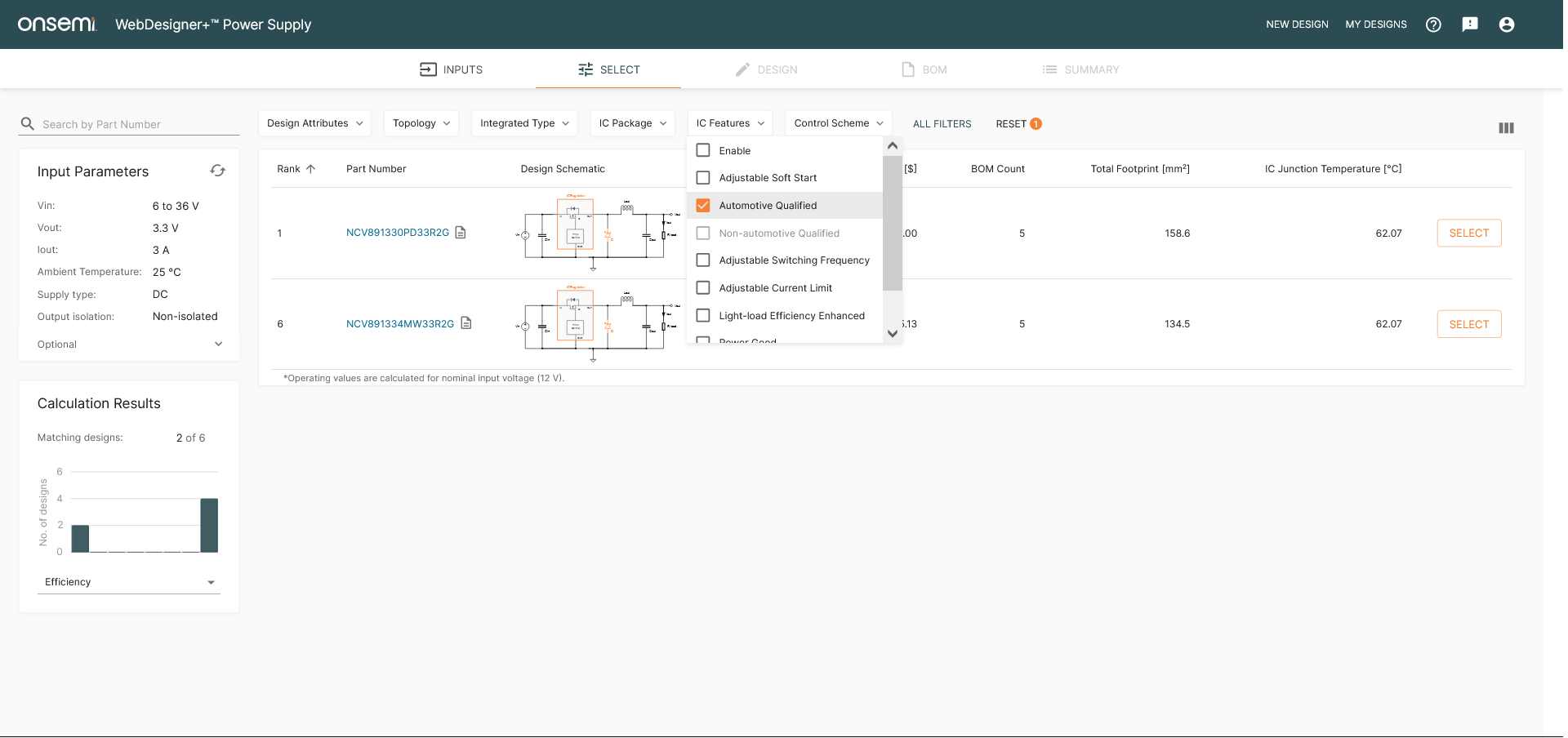Figure 4: SELECT screen of the WebDesigner+ Power Supply tool with solutions filtered for automotive-qualified products only.

You will see SCHEMATIC, OPERATING VALUES, and CHARTS subtabs below the DESIGN tab (see figures 5 and 6). The operating values are listed at the nominal input voltage (12 volts in our example). This is a nod to the designer’s need to be less concerned with operating extremes - other than the fact that the design must survive and function - but be more interested in optimizing performance at the nominal input voltage. Operating values result from the sophisticated mathematical model of the power supply, calculated in an iterative self-consistent manner.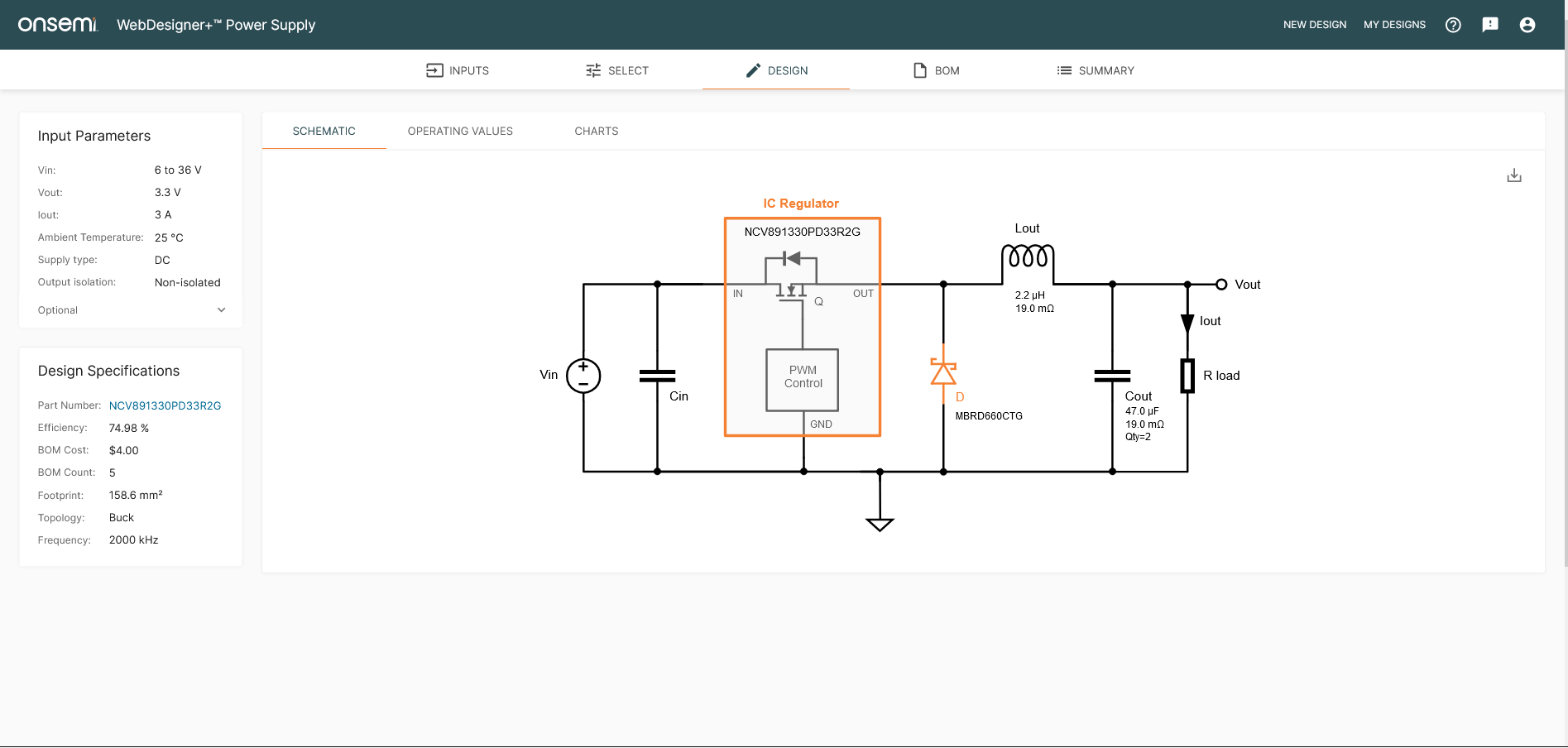Figure 5: DESIGN tab showing the schematic in the WebDesigner+ Power Supply.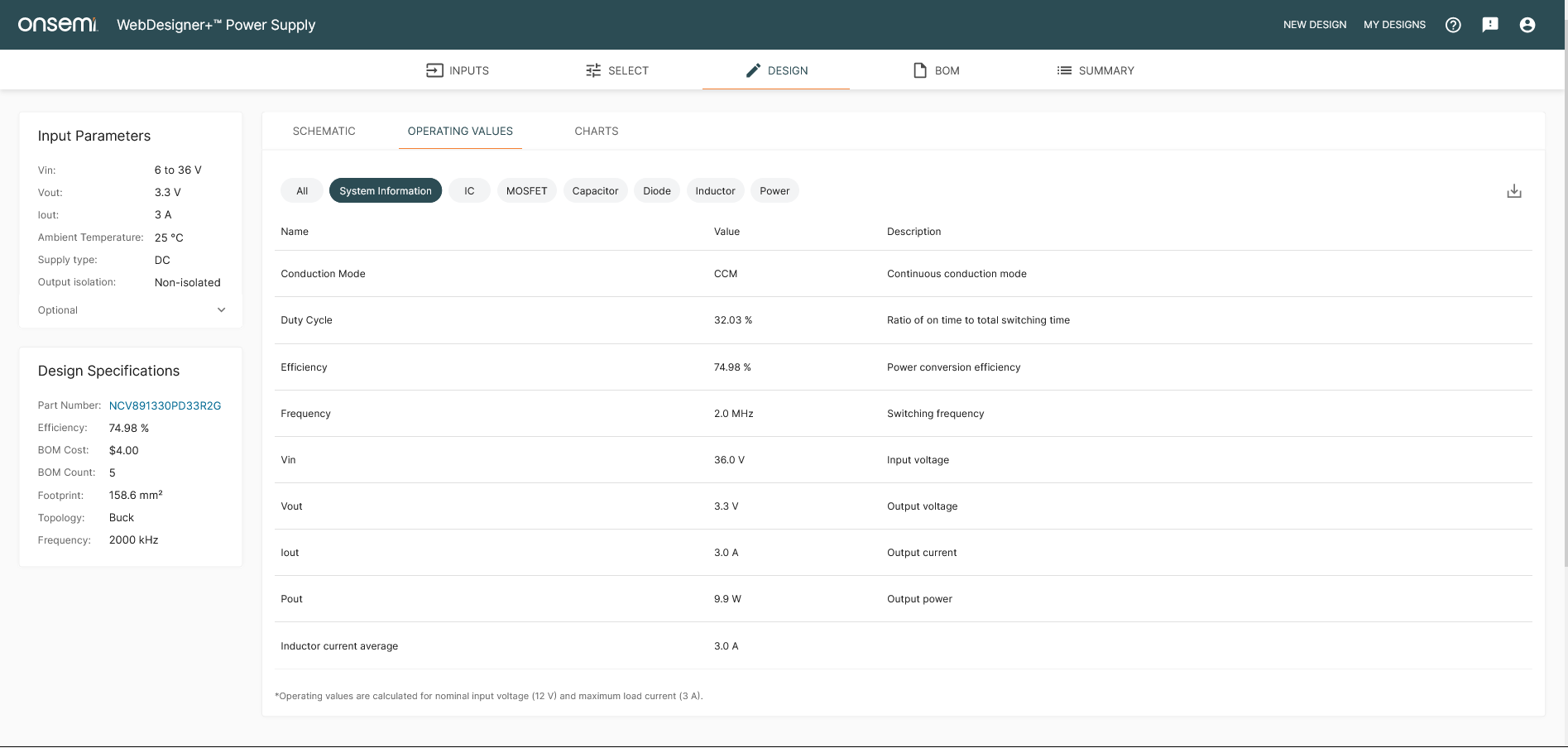Figure 6: DESIGN tab showing the operating values—calculated at the nominal input voltage—in the WebDesigner+ Power Supply.

Click on the CHARTS subtab. In figure 7, one can see that charts plot three input voltage conditions: minimum, nominal, and maximum voltage. Since the maximum and minimum are not symmetrical around the nominal value, the curves are not symmetrical either. Efficiency at maximum input voltage is 65% while 76% at the minimum input; at the nominal value, it is 75%, closer to the input minimum than the maximum. However, as discussed, the designer is not overly concerned with these values since the circuit will spend little time at the extremes. The design spends a predominant portion of the time operating at nominal voltage, giving valuable insight to the designer in choosing their design and focusing on the nominal input voltage.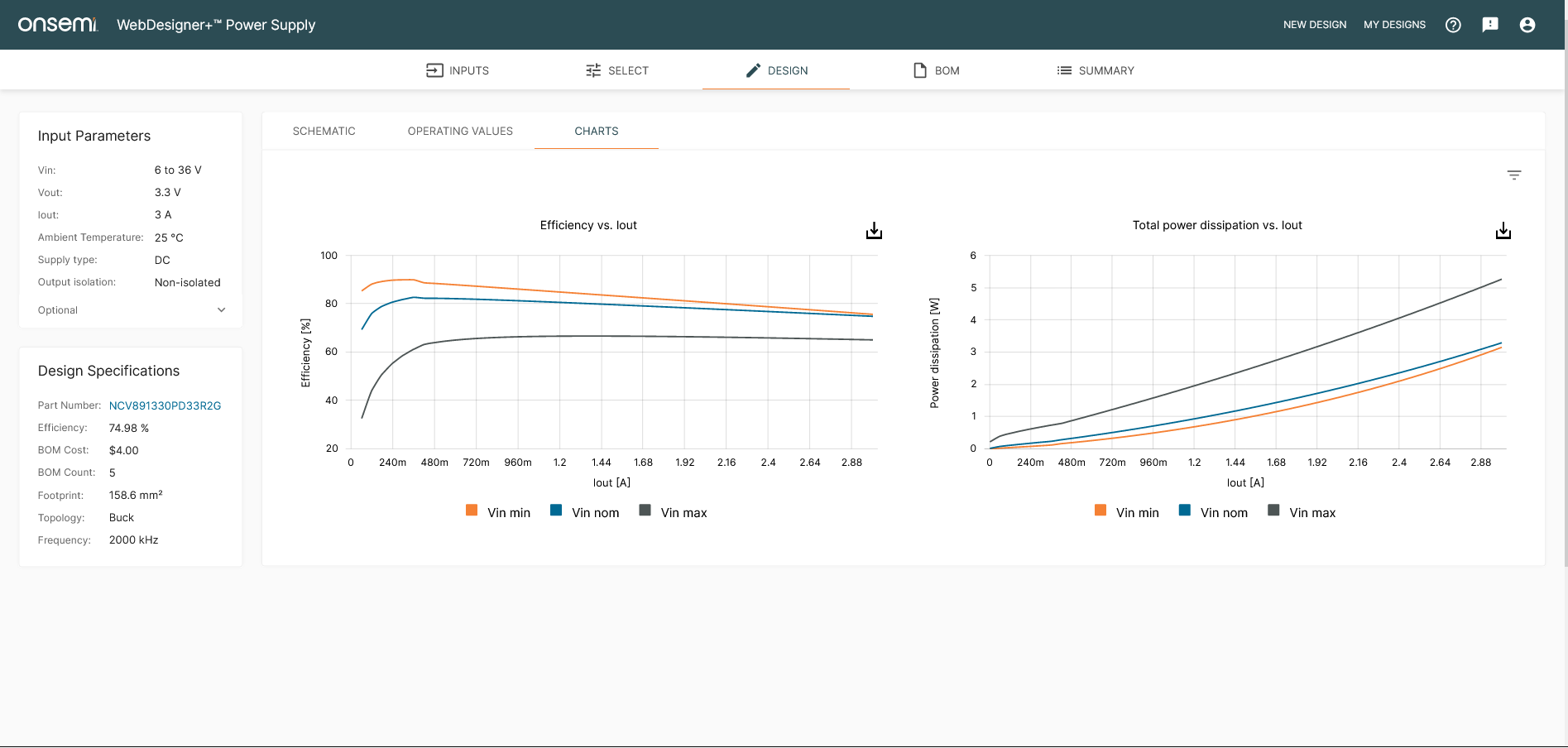Figure 7: DESIGN tab showing the charts in the WebDesigner+ Power Supply. Charts are plotted at the nominal input voltage, in addition to the usual maximum and minimum value.

Suppose the nominal voltage isn’t specified in the INPUT screen. In that case, the charts are calculated at the “nominal” voltage of the average of maximum and minimum (21 V = (3 + 36)/2 volts), and operating values are calculated at the worst case of maximum voltage (36 volts). Neither of these is of particular interest to the user. The SELECT screen ranks designs based on the worst-case (Vin max = 36 volts) performance, not the nominal voltage. As an exercise, you can repeat the above without specifying the nominal voltage to see the difference in results.

Conclusion

Get out of your comfort zone; be normal.

While designing at extremes is vital in some cases, in others it is more important to design for a nominal use case. The next time you design a power supply, you can design for the extremes but optimize for the nominal voltage with the WebDesigner+ Power Supply tool.

For any questions, comments, or assistance on the new WebDesigner+ Power Supply tool, please email us at design.tools@onsemi.com or comment below..blogSocial img{ width: 30px; }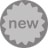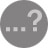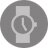0 0 0 0 0

发表评论Connect with us